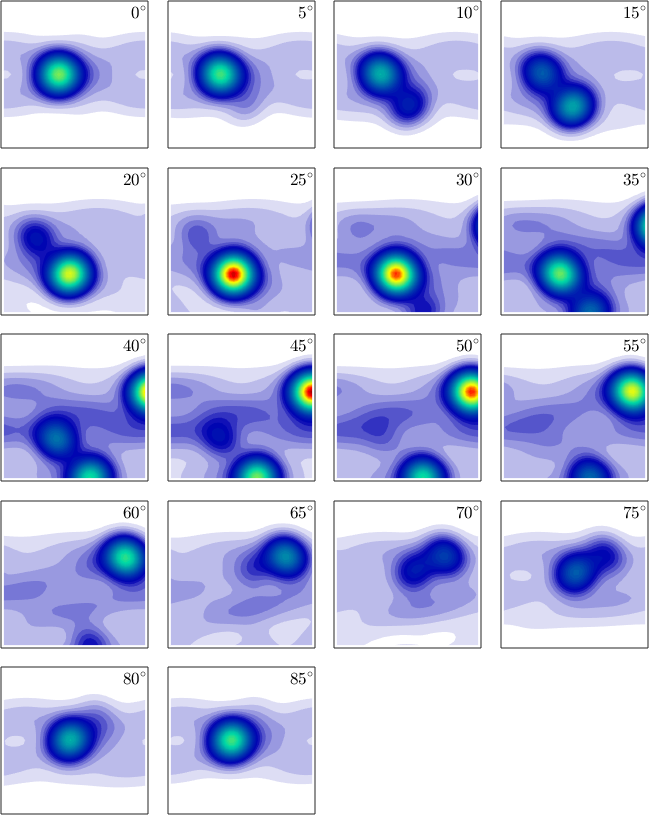Euler Angle Sections edit page

Plotting an ODF in two dimensional sections through the orientation space is done using the command plot. By default the sections are at constant angles of $$\varphi_2$$. The number of sections can be specified by the option 'sections'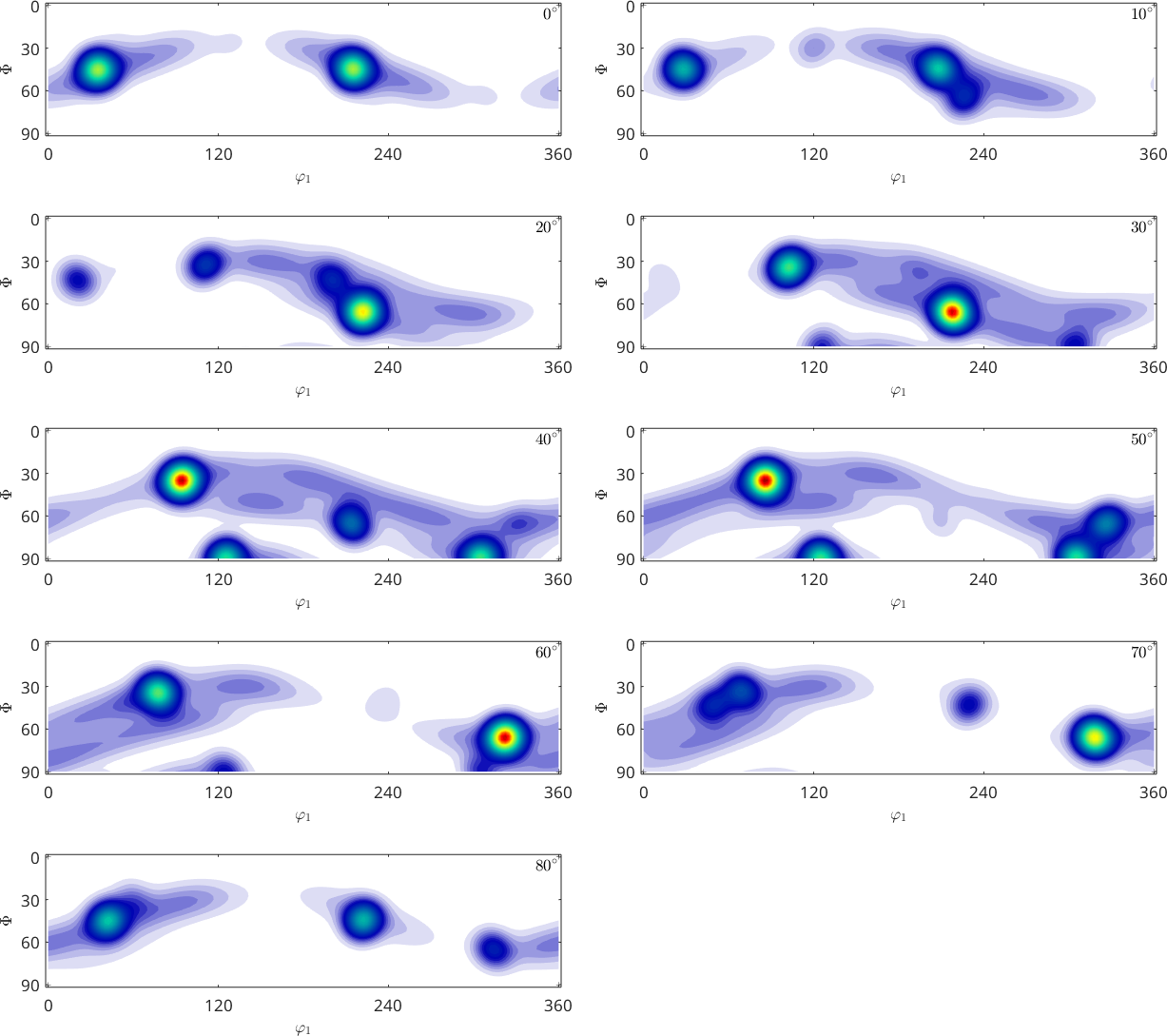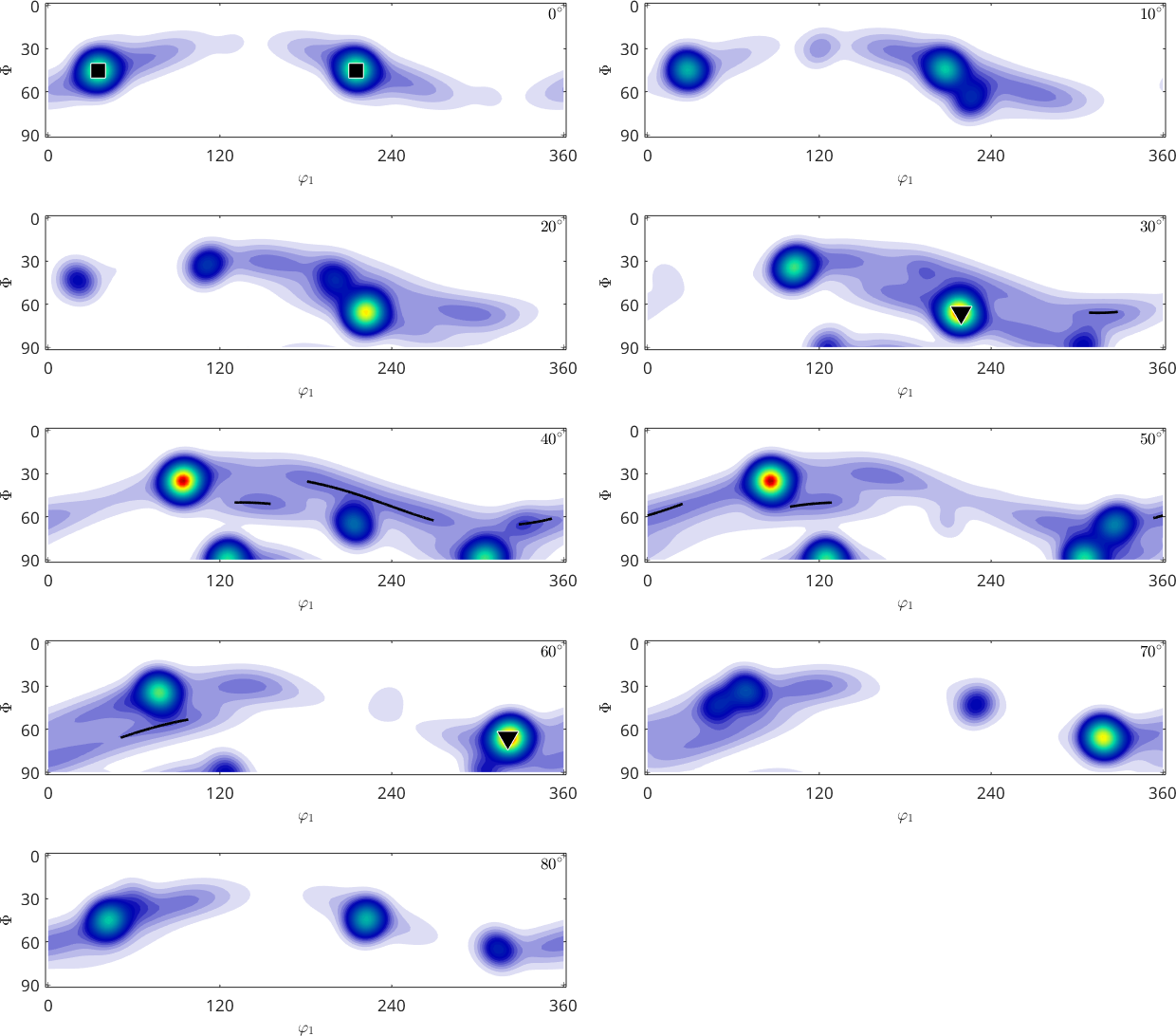One can also specify the $$\varphi_2$$ angles of the sections explicitly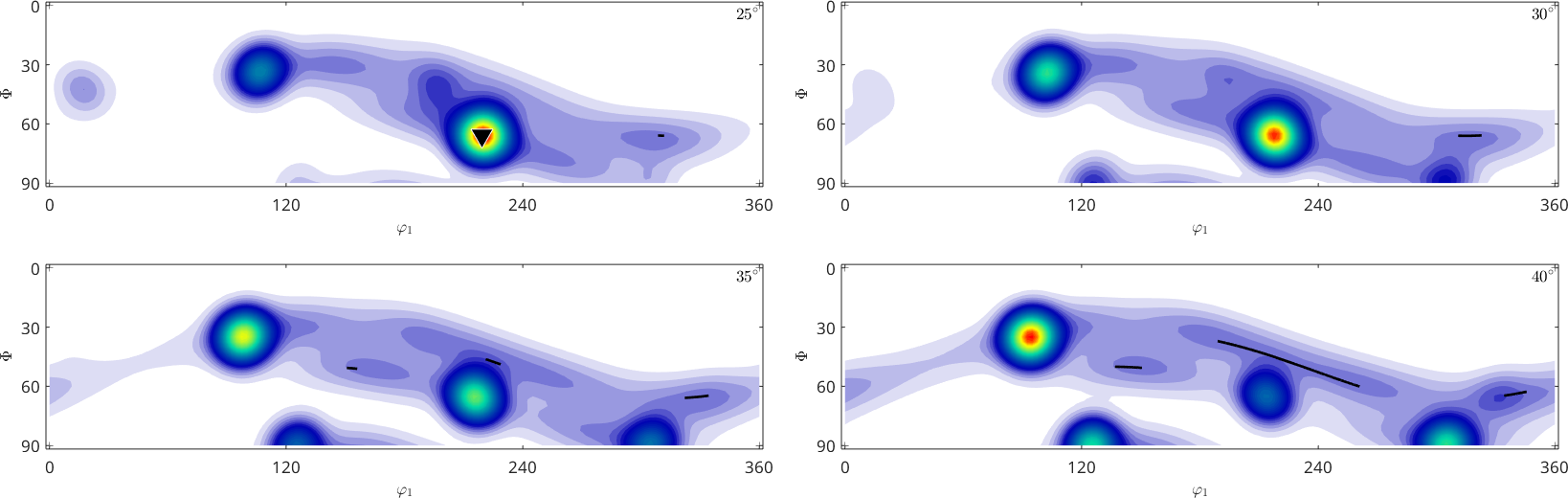Beside the standard phi2 sections MTEX supports also sections according to all other Euler angles.

• phi2 (default)
• phi1
• gamma (Matthies Euler angles)
• sigma (alpha+gamma)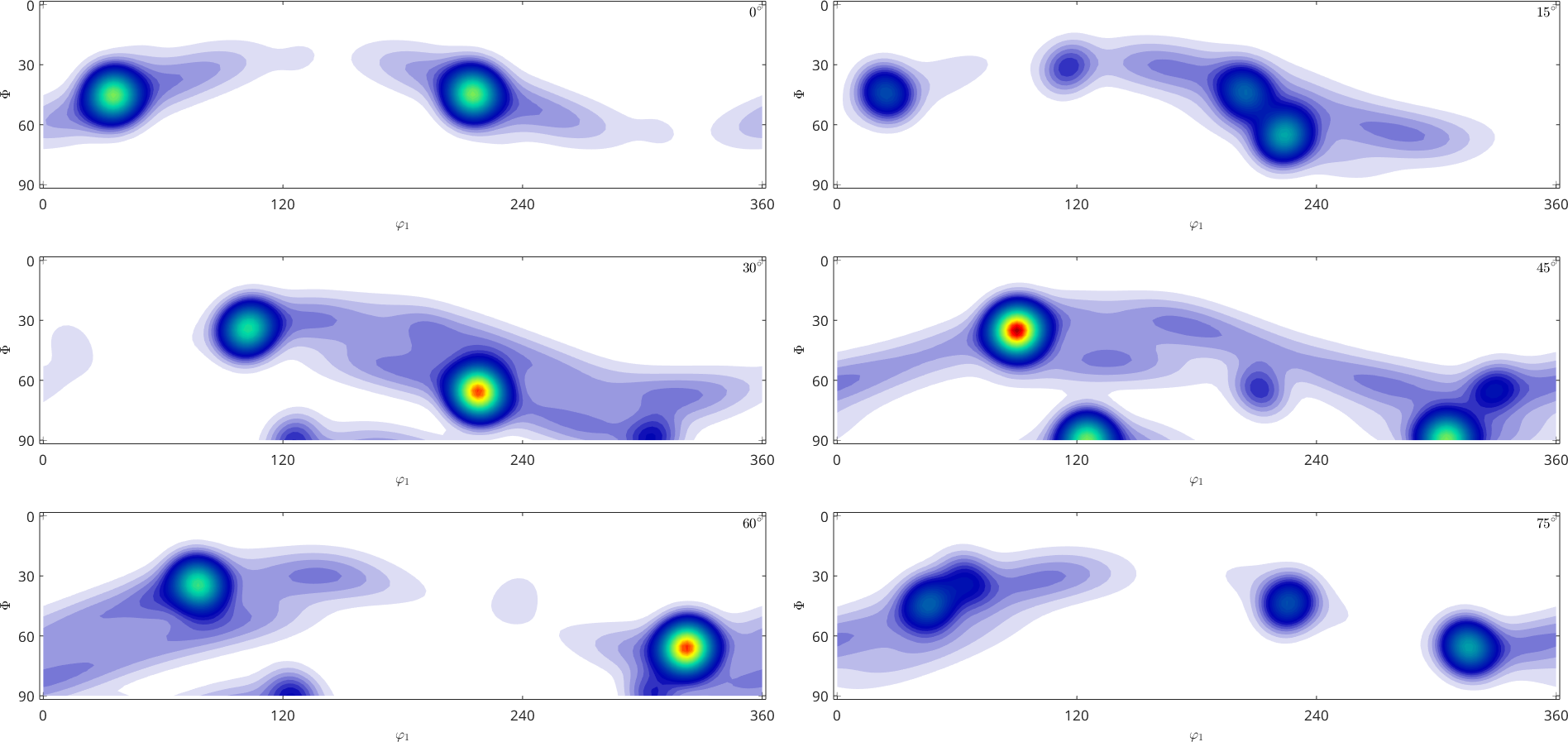By default this command represents the ODF in the Bunge Euler angle space $$\varphi_1$$, $$\Phi$$, $$\varphi_2$$. The range of the Euler angles depends on the crystal symmetry according to the following table

 symmetry 1 2 222 3 32 4 422 6 622 23 432 $$\varphi_1$$ $$360^{\circ}$$ $$360^{\circ}$$ $$360^{\circ}$$ $$360^{\circ}$$ $$360^{\circ}$$ $$360^{\circ}$$ $$360^{\circ}$$ $$360^{\circ}$$ $$360^{\circ}$$ $$360^{\circ}$$ $$360^{\circ}$$ $$\Phi$$ $$180^{\circ}$$ $$180^{\circ}$$ $$90^{\circ}$$ $$180^{\circ}$$ $$90^{\circ}$$ $$180^{\circ}$$ $$90^{\circ}$$ $$180^{\circ}$$ $$90^{\circ}$$ $$90^{\circ}$$ $$90^{\circ}$$ $$\varphi_2$$ $$360^{\circ}$$ $$180^{\circ}$$ $$180^{\circ}$$ $$120^{\circ}$$ $$120^{\circ}$$ $$90^{\circ}$$ $$90^{\circ}$$ $$60^{\circ}$$ $$60^{\circ}$$ $$180^{\circ}$$ $$90^{\circ}$$

Note that for the last two symmetries the three fold axis is not taken into account, i.e., each orientation appears three times within the Euler angle region. The first Euler angle is not restricted by any crystal symmetry, but only by specimen symmetry. For an arbitrary symmetry the bounds of the fundamental region can be computed by the command fundamentalRegionEuler

## Specimen Symmetry

As we can see from the above table the first Euler angle $$\varphi_1$$ ranges for all symmetries from zero to 360 degree. The only way to restrict this angle is to consider specimen symmetry. In the classical case of orthotropic specimen symmetry the range of the first Euler angle reduces to 90 degree and we obtain the common square shaped ODF section plots